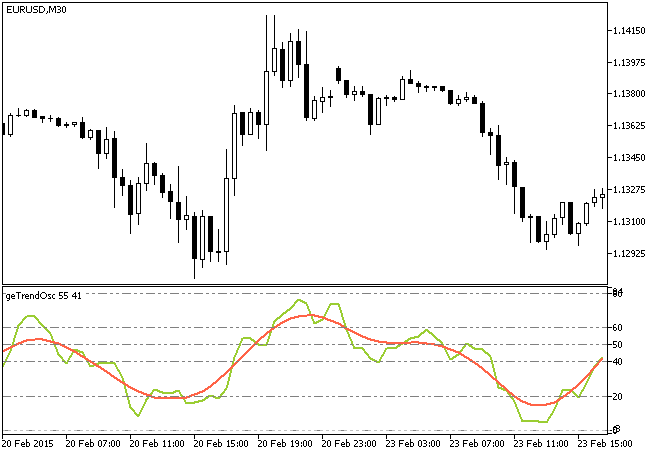Interesting script?
So post a link to it -
let others appraise it

You liked the script? Try it in the MetaTrader 5 terminal# Trend Oscillator - indicator for MetaTrader 5

Views:
4103
Rating:
Published:
2015.04.10 14:12
Updated:
2016.11.22 07:32

Original author:

Evgeniy Gutorov

A trend oscillator indicator. The main algorithm for getting the source signals using the formula:

InputSeries[bar] = (Close[bar] - Open[bar]) / (High[bar] - Low[bar])

To calculate the oscillator signal generated by the green line we use the following formula:

Osc=Sum(Period) / Sum(Abs(Period)) * 100

where:

• Sum(Period) - a sum of source signal values for a period;
• Sum(Abs(Period)) - a sum of absolute source signal values for a period.

The red line is the Hodrick-Prescott filter which redraws if the redraw period in the beginning bars is big. For that reason it's better to use the period of 2 to get more reliable results.

Originally this indicator has been written in MQL4 and was first published in the Code Base on 15.08.2009.Fig. 1 The geTrendOsc trend oscillator

Translated from Russian by MetaQuotes Software Corp.
Original code: https://www.mql5.com/ru/code/12521MAMA_HTF

The MAMA indicator with the timeframe selection option available in the input parameters.Notches

The indicator draws lines by ascending series of maximums and descending series of minimums.Exp_Ozymandias

Trading system using the Ozymandias indicator.Background_FiboCandles_HTF

The indicator draws candlesticks of a larger timeframe as color filled rectangles based on the FiboCandles indicator.